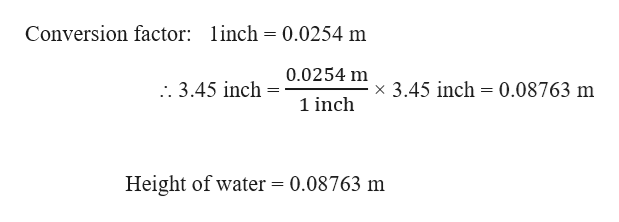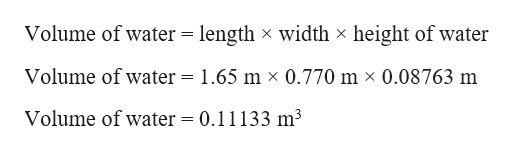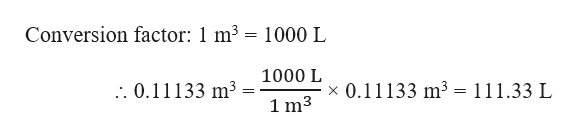A water bath in a physical chemistry lab is 1.65 m1.65 m long, 0.770 m0.770 m wide, and 0.610 m0.610 m deep (high). If it is filled to within 3.45 in3.45 in from the top, how many liters of water are in it?

Question

A water bath in a physical chemistry lab is 1.65 m1.65 m long, 0.770 m0.770 m wide, and 0.610 m0.610 m deep (high). If it is filled to within 3.45 in3.45 in from the top, how many liters of water are in it?

Step 1

Given,

Length of water bath = 1.65 m

Width of water bath = 0.770 m

Height of water bath = 0.610 m

Height of water filled = 3.45 in

Firstly, we have to convert 3.45 inch (in) to meter (m) as follows:help_outlineImage TranscriptioncloseConversion factor: linch = 0.0254 m 0.0254 m x 3.45 inch 0.08763 m . 3.45 inch 1 inch Height of water = 0.08763 m fullscreen
Step 2

The volume of water can be calculated as:help_outlineImage TranscriptioncloseVolume of water = length x width x height of water Volume of water = 1.65 m x 0.770 m x 0.08763 m Volume of water = 0.11133 m3 fullscreen
Step 3

Conversion of m3 to liters (L) can...help_outlineImage TranscriptioncloseConversion factor: 1 m3 = 1000 L 1000 L x 0.11133 m3 = 111.33 L . 0.11133 m3 1 m3 fullscreen

Want to see the full answer?

See Solution

Want to see this answer and more?

Our solutions are written by experts, many with advanced degrees, and available 24/7

See Solution
Tagged in

Chemistry